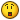## Recommended Posts

Yea idk why this is confusign me so much.

Should of studied harder in math!

I need to convert \$ctime to day/hr/min/sec formatLocal \$hTimer = TimerInit()
Local \$rtime = 2 * 86400000 ;2 days in ms

While 1
sleep(1000)
Local \$fDiff = TimerDiff(\$hTimer)
Local \$ctime = \$rtime - \$fDiff
if \$fDiff > \$rtime Then
;GetnPost()
ToolTip("TIMESUP!!!!",0,0)
Sleep(5000)
Local \$hTimer = TimerInit()
Else

ToolTip("TIMELEFT: " & \$ctime,0,0)
EndIf
WEnd

Thanks for viewing!

Edited by magace

##### Share on other sites

see if this is what you need:

```example1()
example2()

Func example1()
\$miliseconds = 43200000
\$day = (\$miliseconds / 43200000)
\$hour = (\$miliseconds / 3600000)
\$min = (\$miliseconds / 60000)
\$sec = (\$miliseconds / 1000)

MsgBox(4096, "Example 1", "converted milliseconds to day = " & \$day & " day(s)" & _
@CRLF & "converted milliseconds to hour = " & \$hour & " hour(s)" & @CRLF & _
"converted milliseconds to minute = " & \$min & " minute(s)" & @CRLF & _
"converted milliseconds to second = " & \$sec & " seconds(s)")
EndFunc   ;==>example1

Func example2()
\$miliseconds = 49536000
\$day = Int(\$miliseconds / 43200000)
\$hour = Int(Mod(\$miliseconds, 43200000) / 3600000)
\$min = Int(Mod(Mod(\$miliseconds, 43200000), 3600000) / 60000)
\$sec = Int(Mod(Mod(Mod(\$miliseconds, 43200000), 3600000), 60000) / 1000)
\$time = \$day & " day(s) and " & StringFormat("%02d:%02d:%02d", \$hour, \$min, \$sec)

MsgBox(4096, "Example 2", \$time)
EndFunc   ;==>example2```
Edited by Belini

##### Share on other sites```#include <Date.au3>

\$hTimer = TimerInit()
\$rtime = 2 * 86400000 ;2 days in ms

While 1
sleep(1000)
Local \$fDiff = TimerDiff(\$hTimer)
Local \$ctime = \$rtime - \$fDiff
if \$fDiff > \$rtime Then
;GetnPost()
ToolTip("TIMESUP!!!!",0,0)
Sleep(5000)
Local \$hTimer = TimerInit()
Else
ToolTip("TIMELEFT: " & _Convert(\$ctime), 0, 0)
EndIf
WEnd

Func _Convert(\$ms)
Local \$day, \$hour, \$min, \$sec
_TicksToTime(\$ms, \$hour, \$min, \$sec)
If \$hour > 24 Then
\$day = \$hour/24
\$hour = Mod(\$hour, 24)
EndIf
Return StringFormat("%02i/%02i/%02i/%02i", \$day, \$hour, \$min, \$sec)
EndFunc```

## Create an account

Register a new account

• ### Recently Browsing   0 members

×

• Wiki

• Back

• #### Beta

• Git
• FAQ
• Our Picks
×
• Create New...